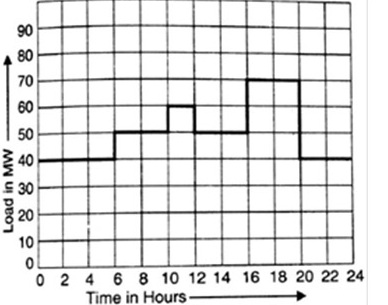A THREE DAY NATIONAL LEVEL ONLINE QUIZ "SKYE -2020 "
DAY-2 (3rd July, 2020) on POWER SYSTEMS - Organised by Department of Electrical and Electronics Engineering,
N.B.K.R Institute of Science &Technology , (Autonomous), Vidyanagar, Nellore (Dt), A.P , Estd. 1979
Name of the participant *
Name of the Institution/Organization *
Name of the Department (In full form i.e ELECTRICAL AND ELECTRONICS ENGINEERING) *
Designation *
Mobile number *
For the given circuit, Ybus and Zbus are bus admittance matrix and bus impedance matrix, respectively, each of size 2×2. Which one of the following statements is true? *
1 point
100% string efficiency means *
1 point
A 75 MVA, 10 kV synchronous generator has Xd =0.4 p.u. The Xd value (in p.u.) is a base of 100 MVA, 11 kV is *
1 point
For a fault at the terminals of a synchronous generator, the fault current is maximum for a *
1 point
A negative sequence relay is commonly used to protect *
1 point
In an inverse definite minimum time, electromagnetic type over-current relay, the minimum time feature is achieved because of *
1 point
In case of transmission line conductors, with the decrease in atmospheric temperature *
1 point
In a 3-step distance protection, the reach of the three zones of the relay at the beginning of the first line typically extends upto *
1 point
The maximum demand of a consumer is 2 KW and the corresponding daily energy consumption is 30 units. What is the corresponding load factor *
1 point
A large hydropower station has a head of 324 m and an average flow of 1370 m3/s. The reservoir is composed of a series of lakes covering an area of 6400 km2. The available hydraulic power is *
1 point
Referring to daily load curve in Fig., the average load is *
1 pointFor a fixed value of complex power flow in a transmission line having a sending end voltage V, the real power loss will be proportional to *
1 point
A load draws a power of 10 kW at a p.f. of 0.707 lagging. If power factor is to be improved to 1, then p.f. correction equipment should supply leading kVAR equal to *
1 point
For the transmission line, if booster transformers are to be used, preferred location will be *
1 point
By increasing the transmission voltage to three times of its original value, the same power can be dispatched keeping the line loss *
1 point